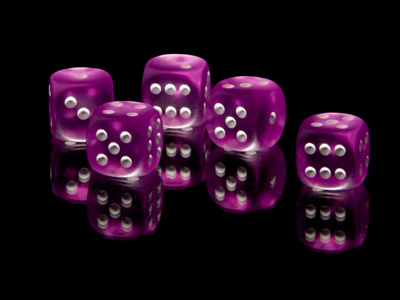The surface area of a cube (such as a dice) with sides of 5cm is 150cm2.

# Perimeter and Area (Year 6)

In KS2 Maths, children learn about perimeter and area. Year Six students figure out the perimeter of shapes like squares, triangles, and rectangles. They also tackle the area of a square, a right-angled triangle, a rectangle, or an L-shape. Converting between mm2, cm2, and m2 becomes a breeze. Now, they explore 3D shapes and how to find their area.

Area is the size of a 2D or 3D shape's surface. For a 3D shape, add the area of each face together. Calculating each face's area is similar to 2D shapes. This Perimeter and Area quiz is all about 2D shapes' perimeter and area, and 3D shapes' surface area.

Take this quiz if you're 10-11 years old and discover what you know about area and perimeter in KS2 Maths.

1.
What is the formula for calculating the area of a rectangle?
2 x length x 2 x width
2 x length + 2 x width
Length + width
Length x width
A rectangle measuring 60cm by 45cm would have an area of 2,700cm2
2.
How would you find the area of an L-shape?
Divide into rectangles; work out the areas of each and then divide one by the other
Divide into rectangles; work out the areas of each and then multiply one by the other
Divide into rectangles; work out the areas of each and then add together
Divide into rectangles; work out the areas of each and then subtract one from the other
Always simplify shapes if you can
3.
What is the perimeter of a regular octagon with sides of 12cm?
20cm
56cm
96cm
160cm
Octagons have 8 sides so 12 x 8 = 96
4.
What is the surface area of a cuboid with measurements of 4cm x 5cm x 6cm?
74cm2
100cm2
148cm2
174cm2
2 of the faces will be 4cm x 5cm = 20cm2
2 of the faces will be 4cm x 6cm = 24cm2
2 of the faces will be 5cm x 6cm = 30 cm2
20 + 20 + 24 + 24 + 30 + 30 = 148
5.
What is the perimeter of a regular decagon with sides of 23cm?
46cm
115cm
200cm
230cm
A regular decagon has 10 equal sides, so we multiply the length of the side by 10 to calculate the perimeter
6.
1cm2 is equivalent to how many mm2?
1mm2
10mm2
100mm2
1,000mm2
There are 10mm in a cm so 10 x 10 = 100
7.
What is the surface area of a cube with sides of 5cm?
25cm2
75cm2
125cm2
150cm2
The area of each face is 25cm2. There are 6 faces, so multiply 25cm2 by 6 to give 150cm2
8.
How do we calculate the area of a right angled triangle?
Length x width then halve
Length + width then double
Length x width then double
Length x width then quarter
Right angled triangles are rectangles cut in half so the formula is the same as for a rectangle but then halved
9.
A regular heptagon has a perimeter of 63cm. What length is each side?
6.3cm
9cm
10cm
12cm
A regular heptagon has 7 equal sides. To find the length of each side divide the perimeter by 7
10.
What is the area of a right angle triangle with width 12cm and length 6cm?
18cm2
36cm2
72cm2
148cm2
12 x 6 = 72
72 ÷ 2 = 36
You can find more about this topic by visiting BBC Bitesize - How to work out an area

Author:  Amanda Swift